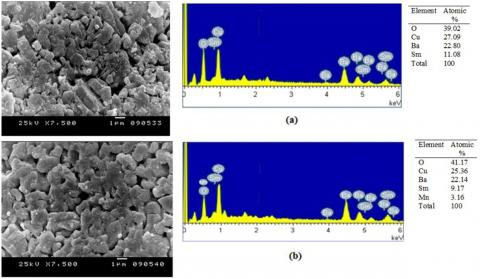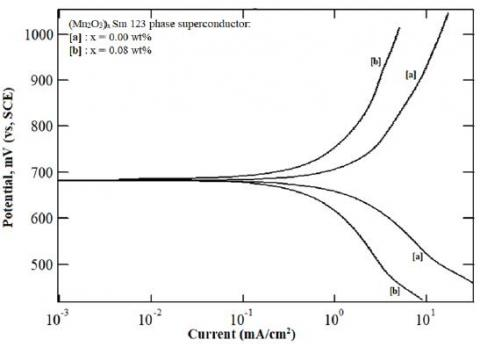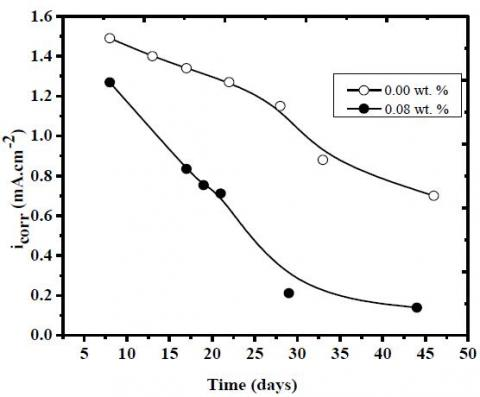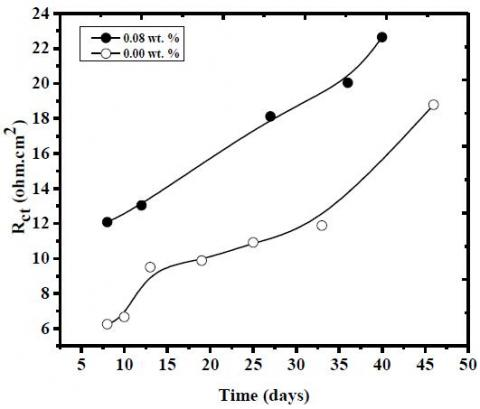# Corrosion Behavior of Mn2O3 Nanoparticles Doped Samarium Superconductor in 0.5 M HCl

Corrosion Behavior of Mn2O3 Nanoparticles Doped Samarium Superconductor in 0.5 M HCl

Department of Chemistry, Faculty of Science, Beirut Arab University, P.O. Box 11-5020 Riad El Solh 11072809 - Beirut, Lebanon

Department of Physics, Faculty of Science, Beirut Arab University, P.O. Box 11-5020 Riad El Solh 11072809 - Beirut, Lebanon

Corresponding Author Email:
ashrafmoustafa@yahoo.com; a.abdelgaber@bau.edu.lb
Page:
195-199
|
DOI:
https://doi.org/10.14447/jnmes.v22i4.a05
27 November 2018
|
Accepted:
25 January 2019
|
Published:
30 December 2019
| Citation
Abstract:

The corrosion behavior of Mn2O3 nanoparticles doped SmBa2Cu3O7-d superconductor, (Mn2O3)x Sm 123, in 0.5 M HCl solutions at 30°C was studied using potentiodynamic polarization curves measurements, and electrochemical impedance spectroscopy (EIS) techniques. Superconducting samples of the type (Mn2O3)xSm 123, were prepared by the conventional solid-state reaction technique. The prepared samples were characterized by scanning electron microscopy (SEM) and energy dispersive X-ray emission spectroscopy (EDX). Results showed that Mn2O3 nanoparticles are positioned at the surface and grain boundaries, reducing the voids present in the Sm 123 matrix. The electrochemical techniques showed that the corrosion current density decreases with increasing the percentages of Mn2O3 nanoparticles and aging time.

Keywords:

corrosion, superconductor, polarization, impedance

1. Introduction

High performance single- domain $(\mathrm{RE}) \mathrm{Ba}_{2} \mathrm{Cu}_{3} \mathrm{O}_{7-5}$ where $\mathrm{RE}$ denoted rare earth element, such as Gd, Nd, Sm, Eu , Y, etc, have received technological and scientific attention since it is used for a wide range of large-scale utilization such as wind turbines, magnetic separation devices for water purification, in transportation as well as in power transmission . Some applications of these new materials will be critically dependent on their stability in hostile aqueous environments which can limit their use in different environments, specifically in humid media . Gao et al.  studied the effect of rare earth elements on the structure and electrochemical properties of $\mathrm{La}_{0.63} \mathrm{R}_{0.2} \mathrm{Mg}_{0.17} \mathrm{Ni}_{3.1} \mathrm{Co}_{0.3} \mathrm{Al}_{0.1}$ alloy electrodes. They found that the substitution of La with Ce, Pr and Gd increases the electrochemical capacity and greatly improves hydrogen diffusion rate in the bulk as well as the charge transfer rate at the surface, and hence increase the rate of discharge-ability. Considerable research effort has been focused on the corrosion resistance of ceramic materials and composite oxides to determine the suitable conditions for different potential applications of these materials and find the protective measures in a corrosive medium. One of the functional methods to enhance the corrosion resistance of these materials is the addition of nanoparticles that became much attractive due to their effect on the microstructure., singh $e t$ al. investigated the Mn-substitution in $\mathrm{Mn}_{\mathrm{x}} \mathrm{Fe}_{3-\mathrm{a}} \mathrm{O}_{4}(0 \leq \mathrm{x} \leq 1.5) .$ It was found that Mn improves significantly the electrocatalytic activity of this material specifically with 0.5 mol Mn-substitution. In addition, the deposition of $\mathrm{MnO}_{2}$ on carbon nanotube (CNT) / Al show that this composite electrode was characterized by its capacitive behavior, displaying a much higher capacitance compared to $\mathrm{MnO}_{2} / \mathrm{Ni}$ electrodes . Furthermore, Wang et al.  showed that the LiNio.sMn $_{1.5} \mathrm{O}_{4}$ cathode material shows the optimal overall electrochemical performance in view of the relatively lower discharge capacity at all rates. Nichelson et al. proved that a maximum ionic conductivity of $10^{-6} \mathrm{S} / \mathrm{cm}$ at ambient temperature is detected for $\mathrm{Li}\left(\mathrm{Li}_{0.05} \mathrm{Ni}_{0.6} \mathrm{Fe}_{0.1} \mathrm{Mn}_{0.25}\right) \mathrm{O}_{2}  .$

In recent years, a lot of attention has been directed to Samarium based superconductor due to its important technological applications. $\mathrm{SmBa}_{2} \mathrm{Cu}_{3} \mathrm{O}_{7-8}$ abbreviated as $\mathrm{Sm}-123$ has been investigated due to its higher critical current density as compared with that of $\mathrm{Y}-123,$ both in low and high magnetic fields $ .$ Therefore, $\mathrm{Sm}-123$ is potentially attractive for high field power applications, static field cooling magnetization  as well as the synthesis of superconducting bulks, tapes, and wires $ .$ Considerable research effort has been directed to the dissolution of $\mathrm{YBaCuO}$ superconductors in aqueous solutions $[11-12] .$ It has been reported that this superconductor is sensitive to water $ .$ However, less attention has been paid to that of $\mathrm{Sm}-123$ superconductors. Consequently, it is interesting to evaluate the influence of added $\mathrm{Mn}_{2} \mathrm{O}_{3}$ nanoparticles to $\mathrm{Sm}-123$ on the corrosion resistance of the superconducting phase.

This work presents a characterization of the $\left(\mathrm{Mn}_{2} \mathrm{O}_{3}\right)_{\mathrm{x}} \mathrm{SmBa}_{2} \mathrm{Cu}_{3} \mathrm{O}_{7}$ o prepared samples and intents to study the effect of the incorporation of $\mathrm{Mn}_{2} \mathrm{O}_{3}$ nanoparticles to $\mathrm{Sm}-123$ superconductor material in $0.5 \mathrm{M}$ $\mathrm{HCl}$ solution at $30^{\circ} \mathrm{C}$.

2. Experimental Techniques

2.1. Working electrode preparation

Superconducting samples of the type $\left(\mathrm{Mn}_{2} \mathrm{O}_{3}\right)_{\mathrm{x}} \mathrm{SmBa}_{2} \mathrm{Cu}_{3} \mathrm{O}_{7-0}$ abbreviated as $\left(\mathrm{Mn}_{2} \mathrm{O}_{3}\right)_{\mathrm{x}} \mathrm{Sm}-123$ were prepared by the conventional solid-state reaction method where $\mathrm{x}=0.00$ to $0.08 \mathrm{wt} . \% .$ High-purity of $\mathrm{Sm}_{2} \mathrm{O}_{3}(99.99 \%), \mathrm{BaCO}_{3}(\geq 99 \%)$ and $\mathrm{CuO}(99.99 \%)$ were purchased from Aldrich chemical company and used in the preparation process. A digital balance was utilized to weigh the appropriate stoichiometric amounts of $\mathrm{Sm}_{2} \mathrm{O}_{3}, \mathrm{BaCO}_{3}$ and $\mathrm{CuO}$ according to the chemical formula of $\mathrm{Sm}: \mathrm{Ba}: \mathrm{Cu}=1: 2: 3 .$ The starting powder was mixed and grounded well in an agate mortar and pestle for about $2-3$ h until a grey powder of the compound was obtained. The resulting powder subjected to the calculation process in air at $900^{\circ} \mathrm{C}$ and $930^{\circ} \mathrm{C}$ for $24 \mathrm{h}$ for each using GALLENKAMP box furnace with intermediate grinding in order to improve the homogeneity of the samples. The resulting powder was grounded and sifted, and then, $\mathrm{x}$ wt. $\%$ of $\mathrm{Mn}_{2} \mathrm{O}_{3}$ nanoparticles was added to the resulting powder and was again mixed to obtain a uniform distribution of nanoparticles throughout the micron powder. The mixed powder was pressed in a disc form $(1.5 \mathrm{cm}$ in diameter and $0.4 \mathrm{cm}$ in thickness) at 14 tons $/ \mathrm{cm}^{2}$ with a hydraulic press, to minimize the intergrain contact problems and to reduce the number of voids in the sample. The pellets were sintered in air at $930^{\circ} \mathrm{C}$ with a heating rate $4^{\circ} \mathrm{C} / \mathrm{min}$ and kept at this temperature for $24 \mathrm{h}$ using horizontal tube furnace (LENTON TYPE LTF $12 / 75 / 610$ ). Then the samples were cooled with a rate $1^{\circ} \mathrm{C} / \mathrm{min}$ to $500^{\circ} \mathrm{C}$ and held at this tem- $-$ perature for $10 \mathrm{h}$ under a flow of oxygen to control the oxygen-content followed by slow cooling at a rate of $1^{\circ} \mathrm{C} / \mathrm{min}$ to room temperature under the flow of oxygen to allow oxygen to be taken up by the sample and provides oxygen stoichiometry close to seven.

## 1.jpgFigure 1. SEM micrographs and EDX spectrum of $\left(\mathrm{Mn}_{2} \mathrm{O}_{3}\right)_{\mathrm{x}} \mathrm{Sm}-123$ phase superconductors (a) $x=0.00$ wt. $\%$ and $(b) x=0.08$ wt. $\%$.

2.2. Solution Preparation

The acid solution $0.5 \mathrm{M} \mathrm{HCl}$ were prepared by dilution of analytical grade acid $37 \%$ HCl purchased from Sigma-Aldrich chemical industries and distilled water were used to prepare the test solutions.

2.3. Samples characterization

The prepared samples were characterized using scanning electron microscopy $(\mathrm{SEM})$ in order to identify the surface morphology and grain connectivity of the samples using a Jeol scanning electron microscope JSM-5300, operated at 25 kV, with a resolution power of 4 nm and a magnification range of $7500 .$ Energy-dispersive X-ray analysis $(\mathrm{EDX})$ was done using detector-type SDD Apollo $\mathrm{X}$ at an accelerating voltage of $20 \mathrm{kV}$ in the interest of studying the atomic composition of the samples.

2.4. Electrochemical studies

Electrochemical impedance (EIS) and polarization measurements were achieved using ACM 1709 potentiostat provided from $\mathrm{ACM}$ instruments (UK). The used cell setup and conditions were comparable to that described previously, in which a saturated calomel electrode (SCE) was used as the reference electrode and a platinum electrode as the auxiliary electrode . The working electrode consists of the prepared superconductor pellets free from and containing different percentages of $\mathrm{Mn}_{2} \mathrm{O}_{3}$ of area $1.893 \mathrm{cm}^{2} .$ Working electrodes were polished, cleaned and left for 40 min to obtain the equilibrium potential in $0.5 \mathrm{M}$ $\mathrm{HCl}$ solution. Polarization curve measurements were done within a $\mathrm{a}$ potential range from $(400 \mathrm{mV})$ to anodic direction $(1000 \mathrm{mV})$ around the rest potential. The frequency ranges of electrochemical impedance spectroscopy (EIS) measurements was 0.01 to $9.6 \times 10^{4} \mathrm{Hz}$ with an applied potential signal amplitude of $\pm 10 \mathrm{mV}$ around the rest potential. Duplicate experiments were performed at $30^{\circ} \mathrm{C}$ in each case of the same conditions in order to test the reliability and reproducibility of the measurements.

3. Results and Discussion

3.1 Samples Characterization

The SEM/EDX results for $\left(\mathrm{Mn}_{2} \mathrm{O}_{3}\right)_{\mathrm{x}} \mathrm{Sm}-123$ phase superconductors with $(\mathrm{a}) \mathrm{x}=0.00 \mathrm{wt} \cdot \%$ and $(\mathrm{b}) \mathrm{x}=0.08 \mathrm{wt} \cdot \% .$ are shown in $\mathrm{Fig} .1$.

The SEM micrographs presented in Fig. $1(\mathrm{a}, \mathrm{b})$ shows a wellconnected plate-like grains randomly oriented in all directions $ .$ Figure 1 (b) shows white particles to superconductor grains explaining that nano-addition does not change the morphological shape of the pure sample, this can be attributed to the sticking of $\mathrm{Mn}_{2} \mathrm{O}_{3}$ nanoparticles to the surface of the grains leading to lower porosity and good connectivity between grains. The percentage of these white particles increase with increasing $\mathrm{Mn}_{2} \mathrm{O}_{3}$ content and are hardly observed in free added sample. In addition, randomly distributed white globules and patches are observed, indicating the formation of $\mathrm{BaCuO}_{2}$ secondary phase . Similar grains morphology was found by Sahoo $e t$ al.  for $Y-123$ added with nano-sized $\mathrm{CoFe}_{2} \mathrm{O}_{4}$ as well as by abdeen et al.$[16-17]$ for $\mathrm{Sm}-123$ added with $\mathrm{ZnFe}_{2} \mathrm{O}_{4}$ and $\mathrm{MnFe}_{2} \mathrm{O}_{4}$.

EDX spectra obtained from the analyses performed in areas with nanometer scale particle entities of $\left(\mathrm{Mn}_{2} \mathrm{O}_{3}\right)_{\mathrm{x}} \mathrm{Sm}-123$ indicate the presence of Mn element implying that $\mathrm{Mn}_{2} \mathrm{O}_{3}$ does not dissolve in the Sm123 structure and stays as adhering material on the grain boundaries leading to fill up of voids and cracks. These results are in good agreement with those obtained by Abdeen $e t$ al.  in $\left(\mathrm{MnFe}_{2} \mathrm{O}_{4}\right)_{\mathrm{x}}$ $\mathrm{SmBa}_{2} \mathrm{Cu}_{3} \mathrm{O}_{7-\delta}$.

3.2 Electrochemical studies

3.2.1 Effect of concentration

3.2.1.1 Potentiodynamic polarization measuremer

The potentiodynamic polarization curves of $\mathrm{Sm}-123$ phase superconductor free from and containing 0.08 wt $\%$ of $\mathrm{Mn}_{2} \mathrm{O}_{3}$ nanoparticles in $0.5 \mathrm{M} \mathrm{HCl}$ is shown in Fig. $2 .$

## 2.jpgFigure 2. Potentiodynamic polarization curves of $\mathrm{Sm}-123$ phase Superconductor free from and containing $0.08 \mathrm{wt} \%$ of $\mathrm{Mn}_{2} \mathrm{O}_{3}$ nanoparticles in $0.5 \mathrm{M} \mathrm{HCl}$ at $30^{\circ} \mathrm{C}$.

As seen, the curves indicate that the incorporation of $\mathrm{Mn}_{2} \mathrm{O}_{3}$ nanoparticles has a slightly effect on the corrosion potential $\left(\mathrm{E}_{\mathrm{corr}}\right) .$ The presence of $\mathrm{Mn}_{2} \mathrm{O}_{3}$ shifts both anodic and cathodic parts of the polarization curves to a lower current value indicating that these nanoparticles retard the corrosion of $\left(\mathrm{Mn}_{2} \mathrm{O}_{3}\right)_{\mathrm{x}} \mathrm{Sm} 123$ superconductors in $0.5 \mathrm{M} \mathrm{HCl}$.

The electrochemical polarization parameters, $\mathrm{E}_{\text {corr }},$ anodic and cathodic Tafel slopes, $\beta_{\mathrm{a}}$, and $\beta_{\mathrm{c}}$, and the corrosion current density, i corr, obtained for $\left(\mathrm{Mn}_{2} \mathrm{O}_{3}\right)_{\mathrm{x}} \mathrm{Sm} 123$ with $(0.00 \leq \mathrm{x} \leq 0.08 \mathrm{wt} . \%)$ are listed in Table 1.

Table 1. Electrochemical polarization parameters obtained for Sm-123 phase superconductor

 Mn2O3 wt.% Ecorr βa βC icorr mV mV.dec-1 mA/cm2 0.00 656.34 376.59 127.54 1.5927 0.02 672.41 326.16 234.07 0.9077 0.04 711.64 289.88 216.58 0.8363 0.06 746.44 257.32 216.35 0.7203 0.08 658.54 392.88 229.32 0.7113

containing different wt.% of Mn2O3 nanoparticles in 0.5 M HCl at 30 ºC.

The tabulated data show that, $\left(\mathrm{Mn}_{2} \mathrm{O}_{3}\right)_{\mathrm{x}} \mathrm{Sm}-123$ phase superconductor free from $\mathrm{Mn}_{2} \mathrm{O}_{3}$ nanoparticles exhibit high corrosion current density that could be attributed to the presence of pores and voids, as shown in figure $1,$ which act as active path that initiate the corrosion process . In addition, increasing $\mathrm{Mn}_{2} \mathrm{O}_{3}$ nanoparticles content decreases the values of $i_{\text {corr }}$ due to the formation of corrosion products over the electrode surface hindering the contact of the surface with the electrolyte, and thus reducing the corrosion rate. The lowest $i_{\text {corr }}$ and highest efficiency is observed for 0.08 wt. $\%$.

3.2.1.2 Electrochemical impedance spectroscopy measurements

The Bode impedance plots of $\mathrm{Sm}-123$ phase superconductor free from and containing 0.08 wt. $\%$ of $\mathrm{Mn}_{2} \mathrm{O}_{3}$ nanoparticles in $0.5 \mathrm{M} \mathrm{HCl}$ are shown in Figures 3.

## 3.jpgFigure 3. Bode impedance spectra of Sm-123 phase superconductor free from and containing 0.08 wt. $\%$ of $\mathrm{Mn}_{2} \mathrm{O}_{3}$ nanoparticles in $0.5 \mathrm{M}$ $\mathrm{HCl}$ at $30^{\circ} \mathrm{C}$.

To analyze the Bode impedance, The intersection of impedance at minimum frequency $\left(Z_{\text {fmin }}\right)$ is the sum of charge transfer resistance $\left.\mathrm{R}_{\mathrm{ct}}\right)$ and solution resistance $\left(\mathrm{R}_{\mathrm{s}}\right) .$ Whereas, that obtained at maximum frequency $\left(Z_{\text {fmax }}\right)$ is equal to the solution resistance $\left(\mathrm{R}_{\mathrm{s}}\right) .$ Therefore, The charge transfer resistance could be calculated using the relation $\mathrm{R}_{\mathrm{ct}}$ $=Z_{\mathrm{fmin}}-Z_{\mathrm{fmax}}[14,15]$.

The values of the electrochemical impedance parameters obtained from the experimental data for corrosion of $\mathrm{Sm}-123$ phase superconductors containing different percentages of $\mathrm{Mn}_{2} \mathrm{O}_{3}$ nanoparticles in 0.5 $\mathrm{M} \mathrm{HCl}$ at $30{ }^{\circ} \mathrm{C}$ are listed in Table 2.

Table 2. Electrochemical impedance parameters obtained for $\mathrm{Sm}-123$ phase superconductor containing different wt.\% of $\mathrm{Mn}_{2} \mathrm{O}_{3}$ nanoparticles in $0.5 \mathrm{M} \mathrm{HCl}$ at $30^{\circ} \mathrm{C}$.

 Mn2O3 wt.% Zfmin Zfmax Rct = Zfmin-Zfmax 0.00 9.13 2.90 6.23 0.02 17.35 2.65 14.70 0.04 19.13 2.83 16.30 0.06 20.90 2.65 18.35 0.08 20.81 2.10 18.71

An increase of $\mathrm{R}_{\mathrm{ct}}$ is observed with nano-addition indicating that $\mathrm{Mn}_{2} \mathrm{O}_{3}$ enhances the corrosion resistance of superconductor phase and retard its corrosion rate in acidic solution. However, the maximum $\mathrm{R}_{\mathrm{ct}}$ value obtained for 0.08 wt.\% $\mathrm{Mn}_{2} \mathrm{O}_{3}$, is in good agreement with the lowest $i_{\text {corr }}$ obtained from polarization measurements and confirming the highest corrosion resistance of $\left(\mathrm{Mn}_{2} \mathrm{O}_{3}\right)_{\mathrm{x}} \mathrm{Sm}-123$ phase superconductors at this concentration. This fact can be explained by the decreasing of the pores diameter and filling up of voids and cracks leading to increase in grain connectivity and reducing the penetration of ionic species into the superconductor material.

3.2.2 Effect of aging time on the stability of the prepared samples

3.2.2.1 Potentiodynamic polarization measurements

The potentiodynamic polarization curves of $\left(\mathrm{Mn}_{2} \mathrm{O}_{3}\right)_{\mathrm{x}} \mathrm{Sm}-123$ (x $=0.08$ wt. $\%$ ) at different aging times are shown in figure 4.

## 4.jpgFigure 4. Potentiodynamic polarization curves of $\mathrm{Sm}-123$ phase superconductor containing $\mathrm{x}=0.08 \mathrm{wt} . \%$ of $\mathrm{Mn}_{2} \mathrm{O}_{3}$ nanoparticles in $0.5 \mathrm{M}$ $\mathrm{HCl}$ at $30^{\circ} \mathrm{C}$ at different time intervals.

It is clearly observed that the potential of $\mathrm{Sm}-123$ shifted to more positive values as the aging time proceeded.

Figure 5 displays the variation of the corrosion current density $i_{\text {corr }}$ obtained from the analysis of the polarization curves versus the aging time for $\mathrm{Sm}-123$ phase superconductor containing $\mathrm{x}=0.00 \mathrm{wt} . \%$ and $0.08 \mathrm{wt} . \%$ of $\mathrm{Mn}_{2} \mathrm{O}_{3}$ nanoparticles.

## 5.jpgFigure 5. Variation of the corrosion current density with aging time for Sm 1233 phase superconductors free from and containing 0.08 wt. $\%$ $\mathrm{Mn}_{2} \mathrm{O}_{3}$ nanoparticles in $0.5 \mathrm{M} \mathrm{HCl}$ at $30^{\circ} \mathrm{C}$.

This figure demonstrate that the pure superconductor sample exhibits the highest current values, which may be related to the presence of diffusion bath for the aggressive media through the voids and pores. A decrease in $i_{\text {corr }}$ was observed for the doped sample, with an aging time up to 30 days, then the stability of corrosion current was reached. This behavior can be attributed to the healing of pits, and voids by the added nanoparticles.

3.2.2.2 Electrochemical impedance spectroscopy measurements

## 6.jpgFigure 6. Variation of charge transfer resistance $\mathrm{R}_{\mathrm{ct}}$ with aging time for $\mathrm{Sm} 1233$ phase superconductors free from and containing 0.08 wt.\% $\mathrm{Mn}_{2} \mathrm{O}_{3}$ nanoparticles in $0.5 \mathrm{M} \mathrm{HCl}$ at $30^{\circ} \mathrm{C}$.

Figures 6 shows the variation of $\mathrm{R}_{\mathrm{ct}}$ obtained from bode impedance plots of $\left(\mathrm{Mn}_{2} \mathrm{O}_{3}\right)_{\mathrm{x}} \mathrm{Sm}-123$ phase Superconductor with $\mathrm{x}=0.08 \mathrm{wt} . \%$ $\mathrm{Mn}_{2} \mathrm{O}_{3}$ nanoparticles in $0.5 \mathrm{M} \mathrm{HCl}$ at $30^{\circ} \mathrm{C}$ at different time intervals.

The obtained results show that the time influences predominantly the corrosion behavior of $\mathrm{Sm}-123$ phase superconductors. It is obvious that the charge transfer resistance $\mathrm{R}_{\mathrm{ct}}$ for $\left(\mathrm{Mn}_{2} \mathrm{O}_{3}\right)_{\mathrm{x}} \mathrm{Sm}-123$ phase superconductor with $\mathrm{x}=0.00 \mathrm{wt} . \%$, and $\mathrm{x}=0.08 \mathrm{wt} . \%$ increases throughout the whole test period, indicating that its corrosion resistance is enhanced as the time elapsed from the sample preparation increase. This may be due to the reducing of the uncovered area available for charge transfer due to corrosion product formation on the phase surface.

In addition, the significant enhancement in $\mathrm{R}_{\mathrm{ct}}$ of the $\left(\mathrm{Mn}_{2} \mathrm{O}_{3}\right)_{\mathrm{x}} \mathrm{Sm}-$ $123(\mathrm{x}=0.08 \mathrm{Wt} \cdot \%)$ superconductor phase, explain the shrinkage of the pores and voids in the doped sample, confirmed by the decrease of i corr obtained from polarization measurements for this sample, at different time intervals.

3.3.3 Corrosion and inhibition mechanism

The enhancement of the corrosion resistance of $\left(\mathrm{Mn}_{2} \mathrm{O}_{3}\right)_{\mathrm{x}} \mathrm{Sm}-123$ phase superconductor to galvanic corrosion is considered as an important issue for studying the corrosion of superconductor phases. Galvanic corrosion is related to the potential of metallic composition of superconductor, electronic characteristics of the nanoparticles, and the aggressive media. In acidic solution, $\mathrm{Mn}_{2} \mathrm{O}_{3}$ nanoparticles are reduced to $\mathrm{Mn}^{2+}$ as described in previous work, $ .$ The standard reduction potentials of $\mathrm{BaO}, \mathrm{Sm}_{2} \mathrm{O}_{3}, \mathrm{CuO}$ and $\mathrm{Mn}_{2} \mathrm{O}_{3}$ are $-2.16,-2,0.62,$ and $1.48,$ respectively $[21-23],$ indicate that $\mathrm{BaO}$ and $\mathrm{Sm}_{2} \mathrm{O}_{3}$ are easily oxidized whereas $\mathrm{CuO}$ and $\mathrm{Mn}_{2} \mathrm{O}_{3}$ are easily reduced in acidic media. Therefore, the higher corrosion rate of $\mathrm{Sm}-123$ is due to the dissolution of $\mathrm{BaO}$ and $\mathrm{Sm}_{2} \mathrm{O}_{3}$. Doping of $\mathrm{Sm}-123$ with $\mathrm{Mn}_{2} \mathrm{O}_{3}$ decreases the percentage of $\mathrm{BaO}$ and $\mathrm{Sm}_{2} \mathrm{O}_{3},$ as clarified from EDX analysis, and hence decreases the corrosion rate. On the other hand, the addition of $\mathrm{Mn}_{2} \mathrm{O}_{3}$ nanoparticles on the surface of the superconductor decreases the voids between the grain boundaries and therefore decrease the solubility of $\mathrm{Sm}-123$ phase superconductor. The reduction product of $\mathrm{Mn}_{2} \mathrm{O}_{3}$ fill up the pores present in the superconductor material leading to decreasing the corrosion rate and corrosion current density to lower values and enhancing the corrosion resistance of $\mathrm{Sm}-123$ phase superconductor in $0.5 \mathrm{M} \mathrm{HCl}$.

4. Conclusion

The following conclusions can be deduced:

1. The electrochemical behavior of $\mathrm{Sm}-123$ phase superconductor can be improved by the addition of $\mathrm{Mn}_{2} \mathrm{O}_{3}$ nanoparticles and preserve their durability in $0.5 \mathrm{MHCl}$.

2. This nano-addition can lead to the decreasing of the pores diameter and filling up of voids and cracks and hence, increasing grain connectivity and reducing the penetration of ionic species into the superconductor material.

3 . $\mathrm{Mn}_{2} \mathrm{O}_{3}$ nanoparticle doped $\mathrm{Sm}-123$ phase superconductor can act as a mixed type inhibitor and can be a promoted material for applications in many fields.

4. The concentration of 0.08 wt. $\% \mathrm{Mn}_{2} \mathrm{O}_{3}$ can be considered as efficient for the enhancement of the electrochemical capacitor applications for $\mathrm{Sm}-123$ phase superconductor.

References

 L.H. Perng, I.C. Tung, and T.S. Chin, Mater. Lett., 29, 265 (1996).

 M. Tonouchi, Y. Sakaguchi and T. Kobayashi, J. Appl. Phys. 27 (1988).

 Z. Gao, X. Zheng, P. Du, and Y. Luo, , J. New Mat. Electr. Sys. 17, 235 (2014).

 N.K. Singh, R. Yadav, and M.K. Yadav, J. New Mat. Electr. Sys. 19, 209 (2016).

 R. Kavian, A. Vicenzo, and M. Bestetti, J. New Mat. Electr. Sys. 18, 43 (2015).

 L. Wang, W. Wu, G. Liu, D. Chen, J. Wang, and G. Liang, J. New Mat. Electr. Sys. 19, 43 (2016).

 A. Nichelson, S. Thanikaikarasan, K. Karuppasamy, S. Karthick-prabhu, T. Mahalingam, X.S. Shajan, and E. Valenzuela, J. New Mat. Electr. Sys. 21, 51 (2018).

 N. Chikumoto, S. Ozawa, S.I. Yoo, N. Hayashi, and M. Murakami, Physica C Superconductivity. 278, 187 (1997).

 R. Weinstein, I.G. Chen, J. Liu, and K. Lau, J. Appl. Phys. 70, 6501 (1991).

 W. Abdeen, A. El Tahan, R. Awad, A.I. Abou Aly, E.M. El-Maghraby, and A. Khalaf, Appl. Phys. A. 122, 547 (2016).

 M.F. Yan, R.L. Barns. H.M. O’Bryan, P.K. Gallagher, R.C. Sher-wood and S. Jin, Appl. Phys. Lett. 15, 532 (1987).

 V.H. Desai and K.B. Sundaram, Phys. Stat. Sol. 143, 109 (1994).

 I.M. Low, S.S. Low and C. Klauber, J. Mater. Sci. Lett. 12, 1574 (1993).

 C. Byoung-Yong, and S.M. Park. Annual Review of Analytical Chemistry. 3, 207 (2010).

 D.V. Ribeiro and J. C. C. Abrantes, Construction and Building Materials; 111, 98 (2016).

 W. Abdeen, A. El Tahan, R. Awad, A. I. Abou Alyl, E. M. El-Maghraby, and A. Khalaf, Appl. Phys. A 122, 574 (2016).

 W. Abdeen, A. El Tahan, M. Roumie, R. Awad, A. I. Abou Aly1, E. M. El-Maghraby, and A. Khalaf, J. Magn. Magn. Mater. 419, 354 (2016).

 M. Sahoo, D. Behera, J. Material. Sci. Eng. 1, 115 (2012).

 H.T. Rahal, A. M. Abdel-Gaber and R. Awad, Int. J. Electrochem. Sci. 12 10115 (2017).

 R. Najjar, A.M. Abdel-Gaber and R. Awad, R. Int. J. Electrochem. Sci, 13 8724 (2018).

 Lide, David R., CRC Handbook of Chemistry and Physics . Boca Raton, FL: CRC Press. ISBN 0-8493-0487-3 (2006).

 A.B.Radwan, R.A.Shakoor and A. Popelka , Int. J. Electrochem. Sci. 10, 7548 (2015).

 P. Vanýsek, "Electrochemical series. Handbook of Chemistry and Physics 93, 5 (2012).